Want to share your content on R-bloggers? click here if you have a blog, or here if you don't.

Categories

1. Visualizing Data

Tags

The PCA (also known as Principal Component Analysis) is quite a handy tool for solving unsupervised learning problems. In other words, PCA can allow us to group unsupervised data into meaningful clusters, and visualize this in a way that allows us to make sense of our data. Let’s see how PCA can be used to analyze traffic patterns.

In this example, we are using traffic data available from the UK Department of Transport website.

Specifically, PCA is used in this instance to analyze traffic routes across London and Edinburgh, and classify routes into different segments based on traffic density.

```setwd("yourdirectory")
summary(myTableedinburgh)
myTableedinburgh
attach(myTableedinburgh)
```

When the dataset is loaded into R, we see that we have the startjunction and endjunction variables: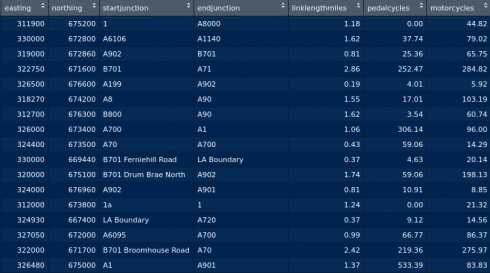We firstly wish to merge these into one variable which gives us both the start and end routes.

```myTableedinburgh\$routes <- paste(myTableedinburgh\$startjunction,myTableedinburgh\$endjunction)
as.data.frame(table(myTableedinburgh\$routes))
```

Now, we can see that the start and end points have been merged under one variable:Specifically, let us assume that we wish to analyze traffic density for buses and coaches. The main thing we are interested in is the frequency of traffic across a particular route.

Let’s take an example. If buses cover 100 miles on a route that is 5 miles long within a certain timeframe, then the frequency will be greater than 100 miles covered on a route that is 10 miles long over the same time period.

We first calculate frequency and then reattach our dataset:

```frequency=busescoaches/linklengthmiles
attach(dataset)
attach(alldata)
```

Now, we must normalize our dataset for the PCA to process it. We will build a function for this purpose:

```#Max-Min Normalization
normalize <- function(x) {
return ((x - min(x)) / (max(x) - min(x)))
}
maxmindf <- as.data.frame(lapply(dataset, normalize))
```

We will fit our data into three clusters and print:

```#ANALYSIS

#pca
column_names<-colnames(maxmindf)
pcamatrix<-scale(maxmindf[,column_names])
pcenter<-attr(pcamatrix, "scaled:center")
pscale<-attr(pcamatrix, "scaled:scale")

#clustering
d<-dist(pcamatrix, method="euclidean")
pfit<-hclust(d,method="ward")
plot(pfit,labels=myTableedinburgh\$routes)
rect.hclust(pfit,k=3)
groups<-cutree(pfit,k=3)

#print clusters
print_clusters<-function(labels,k) {
for(i in 1:k) {
print(paste("cluster",i))
print(maxmindf[labels==i,column_names])
}
}
```

We can now plot our PCA:

```#PCA Plot
library(ggplot2)
pcaoutput<-prcomp(pcamatrix)
numcomponents<-2
cluster=as.factor(groups),
routes=myTableedinburgh\$routes)
p<-ggplot(pcreadings.clusters, aes(PC1, PC2, colour = cluster)) + geom_point() + ggtitle("PCA Analysis") + geom_text(data = pcreadings.clusters, aes(label = routes))
p
```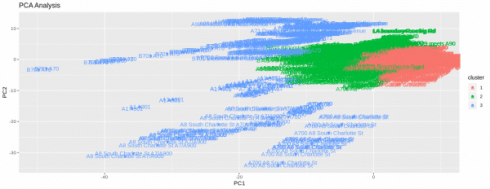We can see that the PCA algorithm has plotted the different routes based on frequency. Let’s take an example.

Here are the miles covered, length of the route in miles, and frequency reading for three sample routes included in three separate clusters:

### Cluster 1: Swanston Avenue to B701 Oxgangs Road### Cluster 2: A6106 to A1140### Cluster 3: A700 to A8 South Charlotte StWe can see that Swanston Avenue to B701 Oxgangs Road has a relatively short road length, along with low traffic and hence low frequency. However, the A6106 to A1140 has a longer road length and higher traffic frequency, while the A700 to A8 South Charlotte Street route has relatively high traffic compared to a short road length, resulting in the highest frequency.

PCA was able to plot this for us intuitively, and thus make it a lot easier to identify routes that are likely to suffer from traffic congestion.

Let’s do the same thing for London.

Again, we are preparing our dataset in the same manner as we did above, and generating a PCA: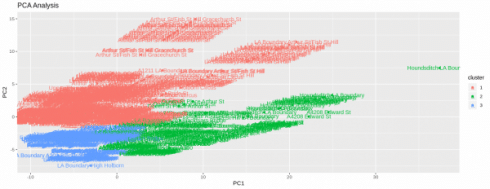Let’s take examples of routes across three different clusters once again:

### Cluster 1: LA Boundary to Holborn Circus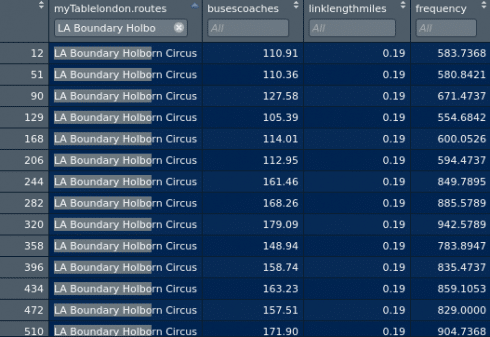### Cluster 2: A3 to London Wall### Cluster 3: Upper Thames Street to A201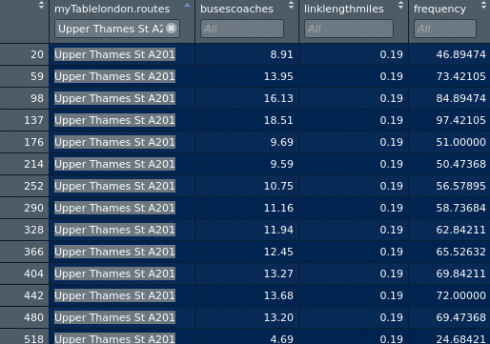Again, we see that the routes across the three different clusters have different characteristics across frequency, length of route and miles traveled within a certain timeframe on the route.

## Conclusion

Here, we have seen how the PCA algorithm has been of use in conducting unsupervised learning on traffic data and identifying areas of high traffic congestion based on the frequency of traffic across a particular route.

Many thanks for your time, and if you are interested in more data science content, please feel free to visit my blog.

Related Post